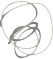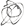Noppanit

20 Mar 2011

# How to find neighbours of 2 dimensional array

So, for example if you have a 2 dimensional array.

1 2 3
4 5 6
7 8 9


the neighbours of “5” is

1 2 3
4 6
7 8 9


the neighbours of “9” would be

5 6
8


Without further ado, here is the algorithm.

private static int[][] array = new int;

private static void initialiseArray() {
int counter = 1;
for (int row = ; row < 3; row++) {
for (int col = ; col < 3; col++) {
array[row][col] = counter;
counter++;
}
}

}

public static void main(String args[]) {
initialiseArray();
for (int row = ; row < 3; row++) {
for (int col = ; col < 3; col++) {
System.out.print(array[row][col]);
if( col == 2 )
{
System.out.println("\n");
}
}
}
neighbours();
}

public static void neighbours()
{
// the position of a cell that you want to display its neighbours
int posX = 2;
int posY = 2;

for( int row = posX - 1; row <= posX + 1; row++)
{
for(int col =  posY -1;  col <= posY + 1; col++)
{
if( !(posX == row &&  posY == col) && row >= && col >= && row < 3 && col < 3 )
{
System.out.println(array[row][col]);
}
}
}

}


Til next time,
noppanit at 00:00